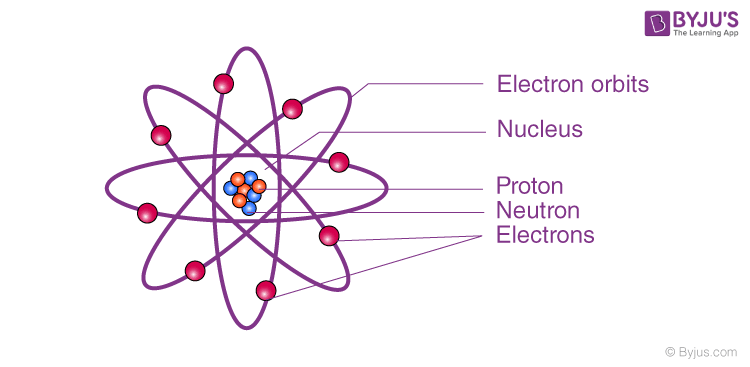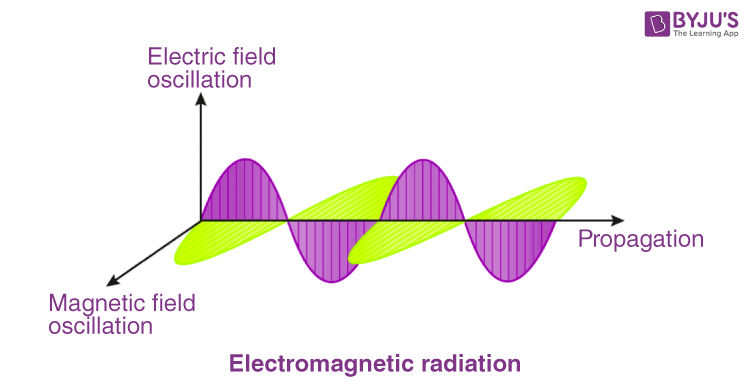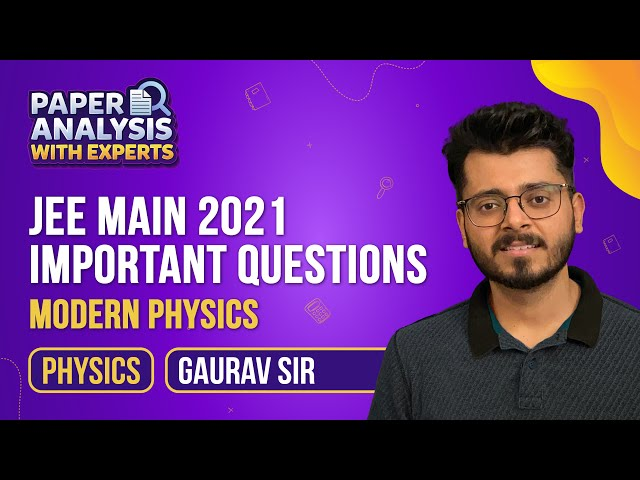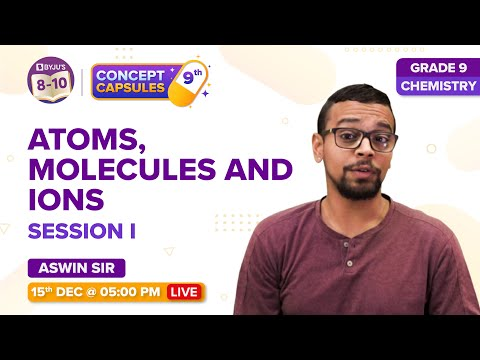# Electrons and Photons

Photons and electrons are two of the basic quantum-mechanical particles but they have completely different properties. Photon is a type of elementary particle which acts as a carrier of energy, but the electron is a subatomic particle which occurs in all the atoms. In this article, we will learn about the definition, meaning and differences between electrons and photons.

## Electron: Meaning, Definition & Formula

The atom comprises three particles: electrons, protons and neutrons. The electron is a subatomic particle that carries a negative charge. Electrons are bound to the atom’s nucleus in, what is called, sub-shells. There are free electrons, too, which are not bound to any atom. Electrons are represented as e or ß. As mentioned earlier, electrons are negatively charged and is measured in Coulomb (the charge of an electron is given as

$$\begin{array}{l}1.60 × 10^{-19}C.\end{array}$$Among the three constituents of an atom, electrons have the lowest mass. The mass of one electron is around 1,800 times less than a proton. If calculated, it comes to 9.10938356 × 10-31 kilograms.

### Electron Wavelength

Louis de Broglie first measured the wavelength of an electron. He used the concepts of Einstein’s formula and Planck’s constant to calculate it. The equation which measures the wavelength of an electron is:

$$\begin{array}{l}\lambda = \frac{h}{p}\end{array}$$

Where,
λ is the wavelength.
h is Planck’s constant.
p is the momentum of the electron.

## Photon: Meaning, Definition & Formula

Photon is a type of elementary particle with a zero rest mass and moves with the speed of light in a vacuum. A photon is the “quantum of electromagnetic radiation”. In other words, it is the smallest and the fundamental particle of electromagnetic radiation. A photon has no mass, no electric charge, and is a stable particle.  These particles possess wave-particle duality. It has 2 polarization states. Photons do not obey Pauli’s Exclusion Principle. Photons are emitted in natural processes. For example, during a nuclear, molecular and atomic transition to a lower level, various photons with different energy levels are emitted, ranging from radio waves to gamma rays. They are also emitted when a particle and its antiparticle are annihilated. The equation E = pc relates the energy and momentum of a photon.

where p is the magnitude of vector p, this comes from the equation

$$\begin{array}{l}E^{2}=p^{2}c^{2}+m^{2}c^{4}\end{array}$$

Photon’s energy and momentum depend on its frequency(v) and wavelength(λ).

$$\begin{array}{l}E=\frac{hc}{\lambda }\end{array}$$

Photon also has a spin angular momentum quantity that does not depend on its frequency.

Light, for example, is electromagnetic radiation. Photons can be said to be the fundamental particles of light. It can be said that light is carried over space by photons.It must be remembered that photons describe the properties of the particles of a wave and not the overall properties of the wave itself. Also, as the photon is essentially nothing but energy, it has no mass whatsoever.

### Difference Between Photon and Electron

Photons and electrons are both fundamental particles, the former of electromagnetic waves and the latter of atoms. Together, they can cause the flow of electricity. However, they are different in many aspects:

 Electron Photon It is a matter. It is energy. It has mass. It has no mass. It carries a negative charge. It doesn’t carry any charge. The speed of an electron can be zero or anything under the speed of light. It is impossible for an electron to achieve the speed of light. Photons travel at the speed of light.

## The video discusses important modern physics questions and its solutions## Get an in-depth understanding of atoms and molecules in this video## Frequently Asked Questions – FAQs

Q1

### What are the three particles which constitutes the atom?

Particles which constitutes the atom are:
• Electrons
• Protons
• Neutrons
• Q2

### What is an electron?

An electron is a subatomic particle that carries a negative charge.

Q3

### Whcih among the constituents of atom has lowest mass?

The electron has the lowest mass.

Q4

### What is the formula to calculate the wavelength of an electron?

$$\begin{array}{l}\lambda = \frac{h}{p}\end{array}$$
Where,

State true or false: Photon travels at the speed of light.
Q5

### State true or false: Photon travels at the speed of light.

True.

Stay tuned to BYJU’S and Fall in Love with Learning!

Test your knowledge on Electrons And Photons